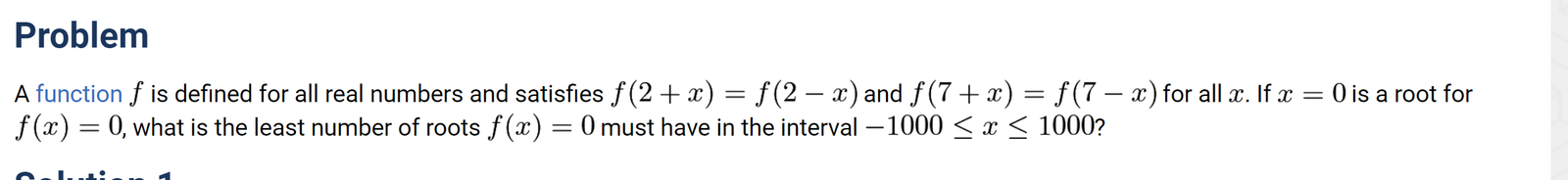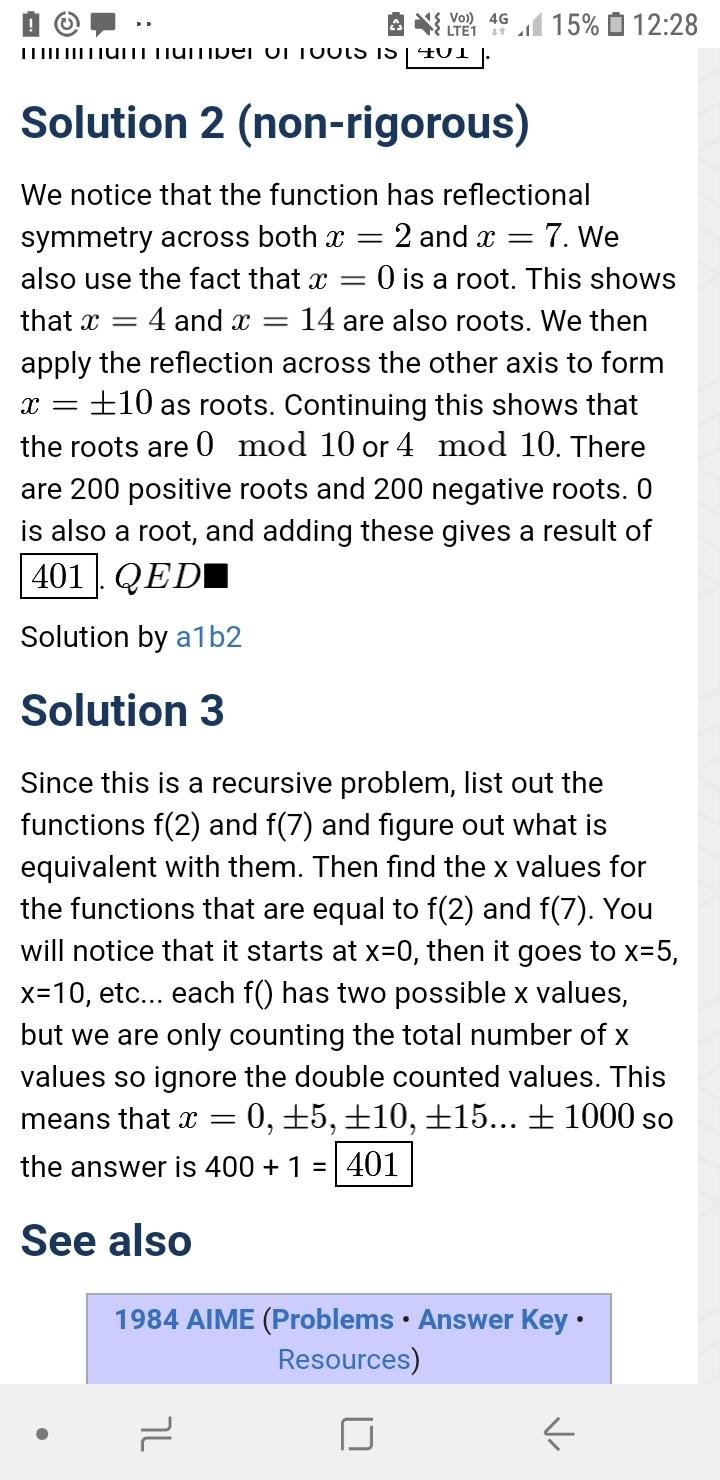# 1984 AIME Problem 12

Home Forums AMC, AIME 1984 AIME Problem 12

Viewing 3 posts - 1 through 3 (of 3 total)
• Author
Posts
• #29180I have seen the solution that was posted in AoPS but I’m still unable to understand.

#29248
1. Show that 4 is a period of f(x) as f(2-x) = f(2+x), put 2-x = y and hence 2+x = 4+y;  f(y) = f(4+y)
2. Show that 14 is a period of f(x) as f(7-x) = f(7+x),put 7-x = z and hence 7+x = 14+z; f(y) = f(14+z)
3. Now f(y) = f(4+y) = f(14+y). This implies that f(y) = f(10+y); i.e. 10 is period. (Put 10+y = t; do the same thing as above.)
4. So, we have got 4,14,10 as period. Now show that 6 is also a period.
5. So we have got 4,14,10,6 as periods. Now show 2 is also a period.
6. Actually what we are doing is that we are applying euclidean algorithm and reaching the gcd of (4,14). In fact you can show that if s and t are the periods of a function, then the gcd(s,t) is also the period and that is the best we can do under the given information.
7. Hence, the minimum period is 2 for the given function.
8. As f(0) = 0, hence due to the periodicity properties f(2) = f(4) =f(6) =… f(1000) = 0 and similarly for the negative side so there are at least 1001 roots.
#29265

On the website, the answer is 401Viewing 3 posts - 1 through 3 (of 3 total)
• You must be logged in to reply to this topic.